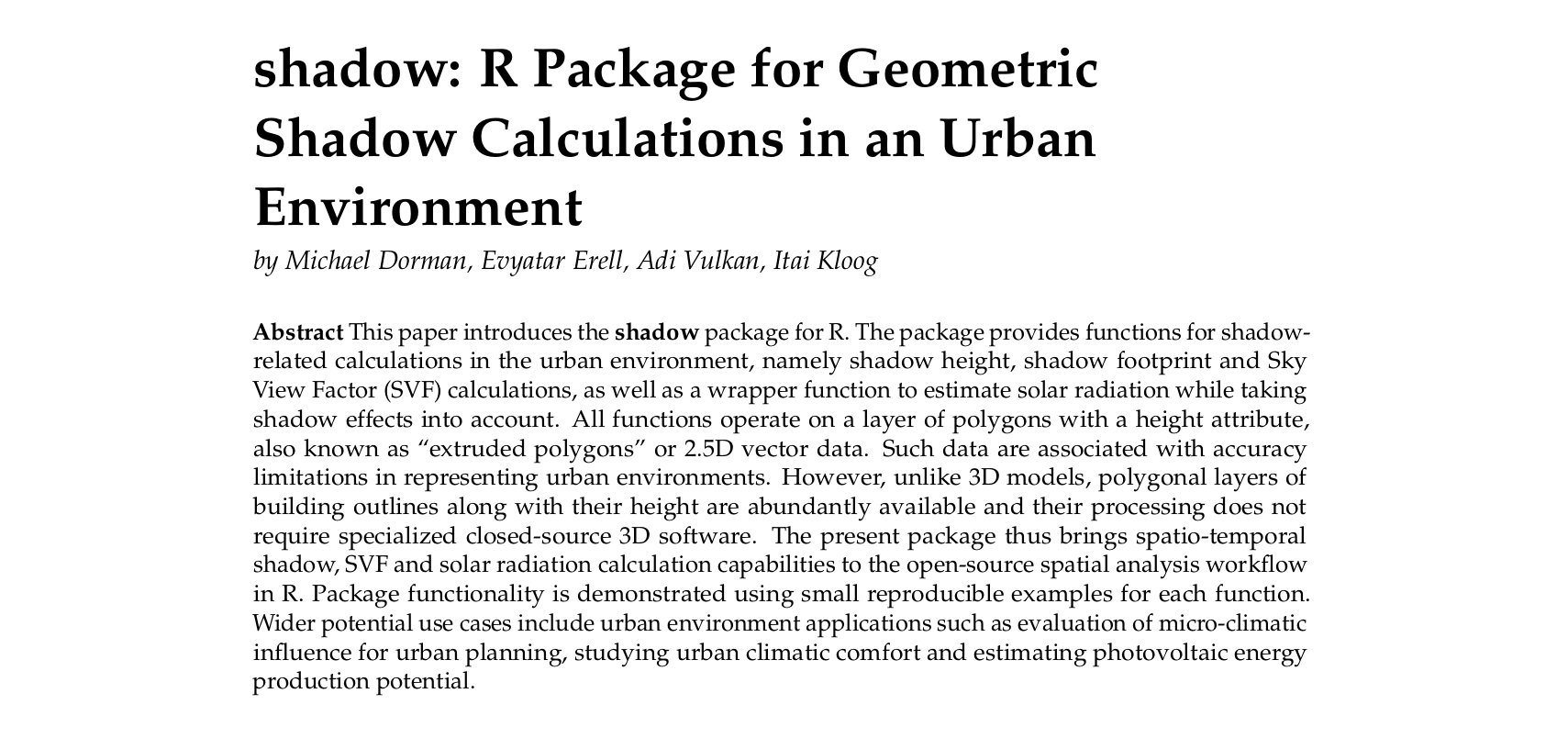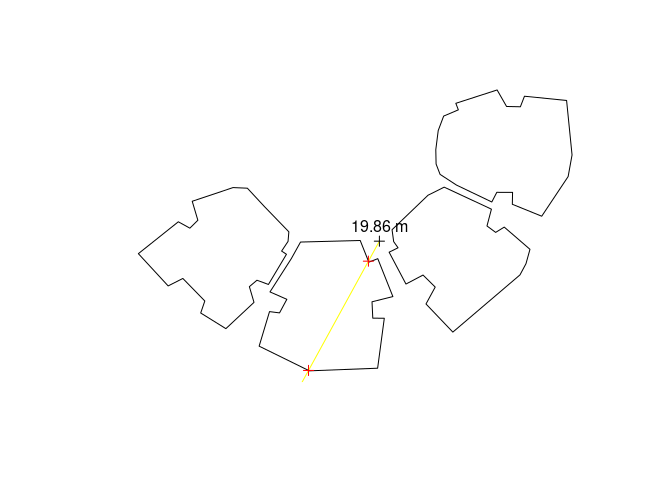### Introduction

shadow is an R package for geometric shadow calculations in an urban environment. A detailed description can be found in the R Journal paper (2019):### Installation

CRAN version:

install.packages("shadow")

GitHub version:

install.packages("remotes")
remotes::install_github("michaeldorman/shadow")

## Documentation

The complete documentation can be found at https://michaeldorman.github.io/shadow/.

### Quick demo

library(shadow)
library(raster)

# Point
location = rgeos::gCentroid(build)

# Time
time = as.POSIXct(
"2004-12-24 13:30:00",
tz = "Asia/Jerusalem"
)

# Location in geographical coordinates
proj4string(location) = CRS("+init=epsg:32636")
location_geo = sp::spTransform(
location,
CRS("+init=epsg:4326")
)
crs(location) = NA

# Solar position
solar_pos = maptools::solarpos(
crds = location_geo,
dateTime = time
)
solar_pos
#>          [,1]     [,2]
#> [1,] 208.7333 28.79944

# Shadow height at a single point
location = location,
obstacles = build,
obstacles_height_field = "BLDG_HT",
solar_pos = solar_pos
)

# Result
h
#>          [,1]
#> [1,] 19.86451

# Visualization
location = location,
sun_az = solar_pos[1, 1],
sun_elev = solar_pos[1, 2]
)
sun_ray = ray(from = location, to = sun)
build_outline = as(build, "SpatialLinesDataFrame")
inter = rgeos::gIntersection(build_outline, sun_ray)
plot(build)
plot(sun_ray, add = TRUE, col = "yellow")
text(location, paste(round(h, 2), "m"), pos = 3)
plot(inter, add = TRUE, col = "red")# Raster template
ext = as(extent(build)+50, "SpatialPolygons")
r = raster(ext, res = 2)

location = r,
obstacles = build,
obstacles_height_field = "BLDG_HT",
solar_pos = solar_pos,
parallel = 2
)

# Visualization
plot(height_surface, col = grey(seq(0.9, 0.2, -0.01)))
plot(location, add = TRUE)